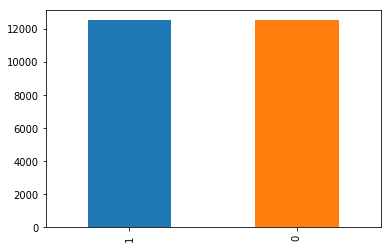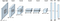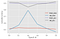# Cats and Dogs classification using AlexNet

In this article, we are going to develop a neural network to classify whether images contain either a dog or a cat using AlexNet architecture. We will use a dataset provided by Kaggle, which contains 25,000 images of dogs and cats.

The distribution of this dataset is shown in the Figure below, where the number 1 represents dogs and number 2 represents cats. From this data, we have 12,500 cats and 12,500 dogs.Figure 1: Distribution of cats and dogs in the dataset.

# AlexNet architecture

AlexNet is a fundamental, simple, and effective CNN architecture, which is mainly composed of cascaded stages, such as convolution layers, pooling layers, rectified linear unit (ReLU) layers and fully connected layers, as shown in Figure 2.Figure 2: AlexNet Architecture

This architecture has five convolutional layers and three fully-connected layers. Besides, RELU and Batch Normalization are applied after every convolutional and connected layer; and Dropout is applied after the first, second, and fifth convolutional layers and the first two connected layer.

The table below shows the sequence of these different layers, its hyperparameters, and the number of parameters.

The equation to find the number of parameters (p) for each stage depends of the layer type:

1. Conv. Layer: It considers the weigth (w) and height (h) of the filter; the number of filters (d) in the previous layer; and the number of filters (k) of the current layer. Thus, we have:

p = (w*h*d + 1) * k

Usually, the weight and height of a filter is the same. Besides, the “+1” factor is related to the bias of the filter.

2. Batch Normalization: In our application, this value will be four times the number of filters.

3. Pooling Layer: It does not have any learning parameter because it only reduces the spatial size of the representation.

4. Fully Connected Layer: It depends of the number of filters in the previous (d) and current (k) layer. Thus, we have:

p = (d+1) * k

Again, the “+1” value represents the bias for each neuron.

It is interesting to observe that in the last fully connected layer shown in Table 1, we have only two classes because in our application we are trying to verify the existence of dogs and cats. In the original implementation of AlexNet, there was 1000 classes, thus, the number of parameters in the last layer is 4096 *1000 = 4096000 parameters in this case.

Finally, we can see that this application has nearly 60 million parameters, which is pretty expensive to train depending on the machine that you are training the NN.

# Training

We divided our entire dataset using as follows: 20,000 images for the training set, and 2,500 images for the validation and test set. Besides, we also used data augmentation to increase the number of images in the training process.

The following hyperparameters were used: Adam optimizer with learning rate of 0.001, L2 regularization with λ=0.0002, batch size = 156, and 55 epochs.

As previously stated, the neural network architecture has nearly 60 million parameters. Thus, the time to train and update all these parameters is very expensive. To not “lose” training information, we will save the weights of the neural network every time when the validation accuracy improves, so that if we want to keep training with more and more epochs at another time, we can load the weights and train the neural network. Besides, each epoch took around 988 seconds or 16 minutes to run! Overall, since we used 55 epochs, we spent around 880 minutes or 14,5 hours to train this neural network! Quite a long time, isn’t it?

In this article, due to the long training time, we decided not to perform any hyperparameter tuning. This configuration will be performed in future work.

Finally, after training 55 epochs, we achieved a validation accuracy of 92.39% and a testing accuracy of 93.68%! These results suggest that the performance of the system improved when using images that it had never seen, suggesting that it is generalizing well. Besides, the system is not overfitting. We could even try to increase the number of epochs, but since it is too computationally expensive, we decided to stop training with 55 epochs.

The figure below resumes the results considering the last 5 epochs, where it is possible to see that 55 epochs achieved the highest value of validation accuracy and the lowest value for the loss.Figure 3: Results of the last 5 epochs.

The code used to generate these results was based on the work developed by Ivanovitch Silva, which can be found here.

# Conclusion

In this article, we studied the neural network architecture called AlexNet, which was developed in 2012, is simple and effective. We applied the AlexNet to classify whether images contain a dog or a cat.

In this application, there are nearly 60 million parameters, which makes the training process computationally expensive. Besides, we trained the model with 55 epochs and it took approximately 15 hours. Therefore, the main limitation of using this architecture is training time. However, using GPU will considerably boost it.

Furthermore, we achieved a testing accuracy of 93.68%, which can be considered very satisfactory for our application. I hope that you have enjoyed and understood the configuration of the AlexNet, a powerful architecture which is very known in the Deep Learning area. See you next time!